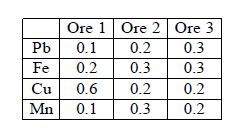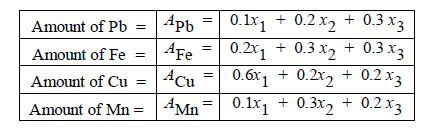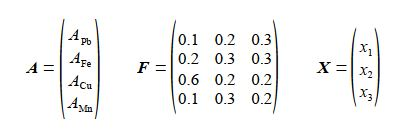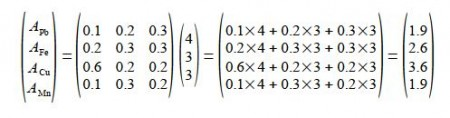# Chemical Ore Analysis

In the lab an analytical Chemist measures the fractional Pb, Fe, Cu and Mn content on a weight for weight basis for three ores, results are shown in below Figure.If the Chemical Ore Analysis are mixed together so there are x1 kg of Ore 1, x2 kg of Ore 2, and x3 kg of Ore 3, the amount of each metal in the mixture can be computed as shown in below Figure.Firstly, rewrite the above series of equations in matrix form A = FX where F is a 4×3 matrix containing the fractional elemental compositions; A and X are column matrices containing the total amount of each element and the quantities of each Chemical Ore Analysis respectively. Secondly, calculate the percent weight for weight (%w/w) of each element in a 10 kg mixture of the ores containing 4 kg of Ore 1, 3 kg of Ore 2 and 3 kg of Ore 3.

Solution

Firstly, the Analytical Chemists results written in matrix form are given below, where A = FX.For the 10 kg of the mixed Chemical Ore Analysis, the product of a 4×3 matrix and a 3×1 matrix is a 4×1 matrix,The 10 kg of mixture has Pb = 19%, Fe = 26%, Cu = 36% and Mn = 19%.